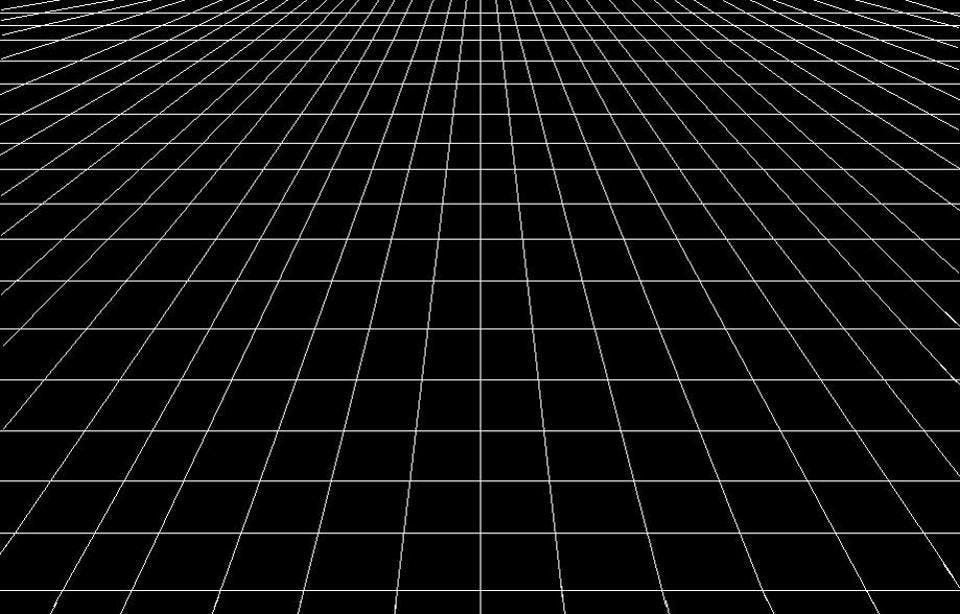# String Theory I: An Introduction

### The Ultimate Goal of Physics

The greatest thing about physics is its ability to describe all phenomena in the universe through simple mathematical equations. These theories can explain all there was, is and will be in the universe. These theories can describe events that occurred billions of years in the past or predict events that will occur billions of years in the future.

But what do we want to achieve? What is the Ultimate goal of physics? Humanity wants to unify all of physics to create a grand theory which contains a “master equation”. This master equation should be able to describe all there is in the universe- the big, the small, the mysterious, the fascinating, all of it. The goal is to answer the “why” and “how” questions. “Why” the universe is the way it is or “how” the laws of physics emerge the way they exist? So far, the closest we have come to this grand theory is String theory.

### The challenge to a grand theory

To create a grand theory of the universe, physicists believe that we have to unify two of the greatest theories humanity has ever created- Quantum mechanics and General Relativity. Seems simple, but it isn’t.

At its most fundamental level, General relativity states that space is flat and has no features. But, in case of Quantum mechanics, we find that space is irregular, violent and filled with disturbances at sub-plack distances (Smaller than Planck length). This is a problem. The theories seem to be incompatible at their most fundamental levels, therefore, cannot be unified.illustration 1. Space according to General RelativityIllustration 2. Space at sub-planck scales according to quantum physics

### How does String Theory solve the problem?

Before the idea of string theory, people tried to unify gravity and quantum mechanics using a theory called the Standard model. The standard model is one of the most successful theories of physics. It predicted the existence and features of many particles known today. But, it failed in one aspect. It could not incorporate gravity.

The Standard Model says that the elementary particles of the universe should be point-like particles. These point-like particles yield mathematical infinities. Infinity equals problem in physics. String theory replaces the notion of point like particles with Strings. Strings are 1 dimensional rubber bands that can vibrate at different frequencies.

To unify gravity and quantum mechanics, string theory solves the problem at the most basic level. String theory “flattens out” the violent nature of quantum space. how? They “ignore” the features of the sub-planck distances. From the perspective of strings, space is flat at all scales. Due to this, String theory promises that it can successfully merge gravity with the quantum world.

### Some properties of Strings

Strings are 10^-35 metres across. This is equal to the Planck Length. Why do strings have such a small size? the answer is tension. the tension in a String is so great – approximately 10^40 newtons – that they are forced to that size.

Strings, as mentioned earlier, vibrate at different frequencies. What do these vibrations mean? String theory says that each frequency of vibration gives rise to a particle with particular properties. The energy of a string depends on the magnitude of frequency of vibration. The greater the frequency, higher the energy. Greater the energy, greater the mass (E=mc^2). Thus the mass of a particle can be determined by vibration of a string. In addition to this, the vibrations of strings also predict the properties of that particle such as charge, spin etc.

For some more strings, follow the series “String theory” on our website and follow our Instagram (@physeeks_made_simple) for interesting content.

## 3 thoughts on “String Theory I: An Introduction”

1.Mrutyunjaya says:

How can we know the length and the force acting on a string? It is made up of energy, right? How can we measure pure energy?

1.Varun Shankar says:

String theory is purely theoretical. We don’t have any experimental measurements or confirmations for string theory as of now. But how can we know whether the values are right? Because we know from the basic properties of strings that certain energies and forces on Strings can give rise to certain kind of particles. If you have any more queries, do ask. Thank you.